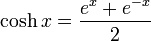# Hyperbolic cosine function

The hyperbolic cosine function denoted$\cosh$, is defined as follows:$\cosh x = \frac{e^x + e^{-x}}{2}$
Here,$e^x$ denotes the exponential function.
More abstractly,$\cosh$ is the even part of the exponential function.